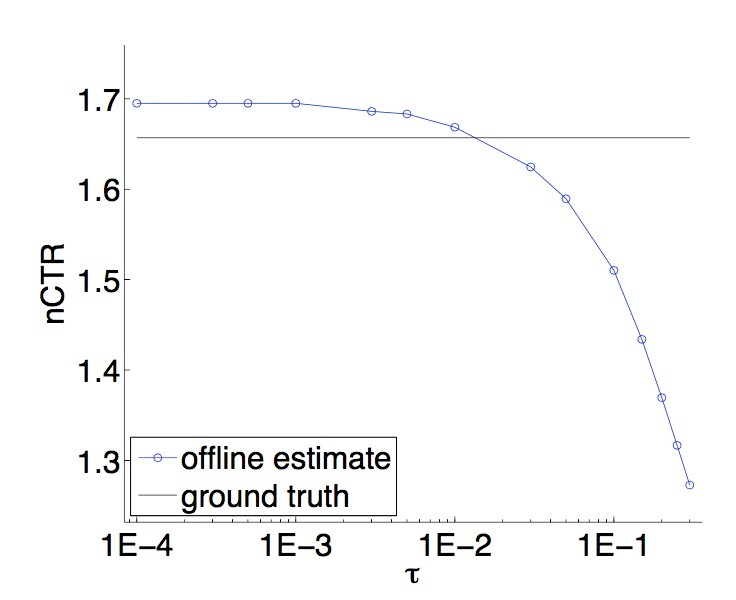介绍

• X是一个任意输入空间
• $A=\lbrace 1, \cdots, k \rbrace$是一个actions集合

• 1.抽取$(x, \vec{r}) \sim D$，并公布x
• 2.算法会选择一个action $a \in A$，可以是一个关于x和历史信息的函数
• 3.公布action a的reward为$r_a$，但不会公布$a'\neq a$是$r_{a'}$

$V^h := \underset{(x,r) \sim D}{E} [r_{h(x)}]$

• 1.监督学习：我们可以学到一个regressor $s: X \times A \rightarrow [0,1]$，它可以在观察到的events上基于action a和其它信息x，训练来预测reward。从该regressor，一个policy可以根据$h(x)=argmax_{a \in A} s(x,a)$来生成。该方法的缺点是，argmax会超出在训练数据中未包含的选择集合，因此不能被泛化。这可以通过一些极端情况进行验证。假设，有两个actions a和b，其中action a出现$10^6$次，action b出现$10^2$次。由于action b的次数比例只占$10^{-4}$，一个学习算法会强迫在预测$r_a$和$r_b$的期望值间做权衡，并压倒性地偏向于估计$r_a$，代价是精准估计$r_b$。然而，在应用上，action b可能会被argmax选中。当action b出现0次时，该问题会更糟糕
• 2.Bandit方法：略
• 3.Contextual Bandits：略
• 4.Exploration Scavenging：略

• 1.对于每个event $(x,a,r_a)$，使用回归(regression)来估计logging policy会选择action a的概率(probability) 为$\hat{\pi}(a \mid x)$。这里，“probability”是随时间变化的——我们可以想像：在不同时间点，采用一个均匀随机的方式从policies集合（可能是deterministic）中抽取。
• 2.对于每个event $(x,a,r_a)$，根据$(x,a,r_a, \frac{1}{max \lbrace \hat{\pi}(a \mid x), \tau \rbrace})$，创建一个synthetic controlled contextual bandit event，其中$\tau > 0$是一些参数。$\frac{1} { max \lbrace \hat{\pi}(a \mid x), \tau \rbrace}$这个量是一个importance weight，它指定了当前event对于训练是有多重要。需要明确的是，参数$\tau$对于数值稳定性很重要。
• 3.应用一个offline contextual bandit算法到synthetic contextual bandit events集合上。在我们第二个实验结果的集合（4.2节）中，采用的argmax regressor的变种使用了两个关键修改之处：(a) 我们将argmax的范围限定在具有正概率的那些actions上 (b) 我们对events进行importance weight，以便训练过程会强调对于每个action平等的好估计。需要强调的是，在本paper中的理论分析可以应用于对于在contextual bandit events学习上的任何算法——我们选择该方法是因为它很容易在已存在方法上进行简单修改。

• 1.在当给定特征x时，logging policy会确定式(deterministically)选中一个action (ad) a，$\hat{\pi}(a \mid x)$意味着什么，？基本observation：一个policy如果在day 1会确定式选中action a、接着在day 2会确定选中action b，这可以被看成是：(当events数目在每天都相同时，并且events间是IID的），在action a和b间使用概率0.5进行随机化。因而，在logged events的时间跨度上，$\hat{\pi}(a \mid x)$是一个给定特征x关于action a被展示的期望频率的估计。第3节中，我们会展示该方法在期望上是合理的，它提供了关于new policy的值的一种无偏估计。
• 2.在$\hat{\pi}(a \mid x)$上的客观误差（inevitable errors）是如何影响该过程的？结果表明它们有影响，取决于$\tau$。对于非常小的$\tau$值，$\hat{\pi}(a \mid x)$的估计必须极其精准来生成好的效果；而对于更大的值，会需要更小的accuracy。第3.1节会证明健壮性
• 3.参数$\tau$是如何影响最终结果的？当在估计过程中创建一个bias时，结果表明该bias的形式是很轻微的并且相对合理的——基于条件x具有低频展示的actions具有一个under-estimated value。这与期望的对于没有频率的actions的限制是一致的。

2.问题设定和假设

• $\pi_1, \cdots, \pi_T$是T个policies，

• $(x,r)$按第1节所述的方式从D中抽取
• action $a \sim \pi_t(x)$根据第t个policy被选中。

offline policy estimator

$S = \lbrace (x_t, a_t, r_{t,a_t}) \rbrace_{t=1}^T$

…(1)

$\hat{V}_{\hat{\pi}}^h(S) = \frac{1}{|S|} \sum\limits_{(x,a,r) \in S} \frac{r_a I (h(x)=a)}{max \lbrace \hat{\pi}(a \mid x), \tau \rbrace}$

…(2)

3.理论成果

• 1.我们具有一个policy estimation阶段，其中我们估计未知的logging policy;
• 2.我们具有一个policy optimization阶段，其中我们会使用我们估计的logging policy。

logging policy $\pi_t$可能是确定的(deterministic)，这意味着：基于logging policy中的随机化的常用方法是不可用的。我们会在下面展示：当现实情况是IID条件、并且policy会随actions的不同而变化时，这是ok的。我们会有效使用算法中的随机化标准方法来替代现实中的随机

$\pi(a | x) = \underset{t \sim UNIF(1,\cdots,T)}{E} [\pi_t (a | x)]$

…(3)

stochastic policy $\pi$会在T policies $\pi_t$上随机均匀选择一个action。我们的第一个结果是，当现实中根据$\pi$或者policies序列$\pi_t$进行选择actions时，我们的estimator的期望值与是相同的。尽管该结果和它的证明是简单的，它是本paper后面部分的基础。注意，policies $\pi_t$可以是任意的，但我们会假设，它们不依赖于用于evaluation的数据。该假设只对证明来说是必要的，可以在实际中放宽些，如4.1节。

$E_{S \sim (D,\pi_i(\cdot|x))_{i=1}^T} \hat{V}_{\hat{\pi}}^h(S) = E_{(x,\vec{r}) \sim D, a \sim \pi(\cdot |x)} \frac{r_a I (h(x)=a)}{max \lbrace \hat{\pi}(a|x), \tau \rbrace}$

…(4)

S就是上面指的dataset。

3.1 Policy Estimation

$reg(x)= \underset{a \in A}{max} [(\pi(a \mid x) - \hat{\pi}(a \mid x))^2]$

…(5)

• $I(\cdot)$表示了indicator function
• $\pi(a \mid x)$是logging policy在输入x上选择action a的概率
• $\hat{V}_{\hat{\pi}}^h$是由等式(2)基于参数$\tau$的定义

• $\hat{\pi}$是从X到在actions A分布上的任何函数
• $h: X \rightarrow A$是任意的deterministic policy。
• $V^h(x) = E_{r \sim D(\cdot \mid x)}[r_{h(x)}]$表示在input x上执行policy h的期望值

$E_x [ I(\pi(h(x) | x) \geq \tau) \cdot (V^h(x) - \frac{\sqrt{reg(x)}}{\tau}) ] \leq E[\hat{V}_{\hat{\pi}}^h] \leq V^h + E_x [ I(\pi(h(x) \mid x) \geq \tau) \cdot \frac{\sqrt{reg(x)}}{\tau} ]$

$E_x [ I(\pi(h(x) | x) \geq \tau) \cdot V^h(x)] \leq E[\hat{V}_{\hat{\pi}}^h] \leq V^h$

$E[\hat{V}_{\hat{\pi}}^h]=\pi(a_1 \mid x) / \hat{\pi}(a_1 \mid x) = (\tau + \epsilon) / \tau$

$E[\hat{V}_{\hat{\pi}}^h] - V^h = |(\tau+\epsilon)/\tau - 1| = \epsilon /\tau$

$(\pi(a_1 \mid x) - \hat{\pi}(a_1 \mid x))^2 = \epsilon^2$

3.2 Policy Optimization

$\pi(a | x) = E_{t \sim UNIF(1,\cdots,T)}[\pi_t(a | x)]$

3.1节的结果可以应用到policy optimization问题上。然而，注意，该数据被看成是通过从一个关于T个policies $\pi_i, \cdots, \pi_T$的执行序列上抽取得到，而非从$\pi$上进行T个抽取得到。

• $\hat{\pi}$是任意从X到actions A分布的函数
• H是任意deterministic policies的集合

• $\tilde{H}=\lbrace h \in H \mid \pi(h(x) \mid x) > \tau, \forall x \in X \rbrace$
• $\tilde{h} = argmax_{h \in \tilde{H}} \lbrace V^h \rbrace$

• $\hat{h} = argmax_{h \in H} \lbrace \hat{V}_{\hat{\pi}}^h \rbrace$是最大化在等式(2)中定义的empirical value estimator的hypothesis。

$V^{\hat{h}} \geq V^{\tilde{h}} - \frac{2}{\tau} (\sqrt{E_x[reg(x)]} + \sqrt{\frac{ln(2|H|/\delta)}{2T}})$

…(6)

4.评估

4.1 实验I4.2 实验II

$\hat{V}_{\hat{\pi}}^h = \frac{1}{T} \sum\limits_{t=1}^T \frac{y_t I(h(x_t)=a_t)}{max \lbrace \hat{\pi}(a_t | x_t) , \tau \rbrace}$

…(7)

Interval列的计算使用Chernoff bound相对熵的形式，$\delta=0.05$持有假设：变量是IID的，（即：在我们的case中，用于estimator计算的样本）。注意，该计算有些复杂，因为变量的范围是$[0, 1/\tau]$，而非常见的$[0, 1]$。这可以通过乘以$\tau$来进行缩放，应用到bound上，接着将结果乘以$1/\tau$进行缩放。

“random” policy指的是，从feasible ads集合中随机选择：$Random(x)=a \sim UNIF(C(X))$，其中$UNIF(\cdot)$表示均匀分布。

“Naive” policy对应于理论上有缺陷的监督学习方法（在介绍中详细说过）。这种policy的evaluation相当昂贵，需要每个example每个ad进行一个evaluation，在此，test set的size被减小到一个click 8373个examples，这会减小结果的重要性。通过按时间先后顺序选择在test set中的第一个events（例如：在training set中与它们最相似的events），我们会将该结果偏向于naive policy。然而，naive policy会收到0 reward，这要比其它方法都要小很多。使用这种evaluation的一个可能担忧之处是，naive policy总是会找到那些不会被explored的good ads。一种快速验证表明：这是不正确的——naive argmax会简单地做出难以置信的选择。注意，我们只上报了evaluation against $\tau=0.05$，因为evaluation against $\tau=0.01$并不明显，尽管reward仍是0.

“Learned” policies会依赖于$\tau$。如定理3.2所示，随着$\tau$是减小，我们相竞争的hypotheses的有效集合是增加的，从而允许learned policy具有更好的效果。确实，当我们把$\tau$从0.05减小到0.01时，learned policy和random policy的估计会同时提升。

empirical结果与理论方法大致是consistent的——它们提供了一个一致的关于policy value的最坏估计，不过它们具有足够动态的范围来区分learned polices vs. random policies，以及learned policies在更大空间(更小$\tau$)vs.更小空间(更大$\tau$)上，理论上不完善的naive方法 vs 合理的方法(在ads的explored空间上进行选择)。

参考

Updated on

STAR算法介绍

PLE介绍

Published on March 04, 2021

DTS介绍

Published on January 02, 2021